# 一、图像处理预备知识

## 2、图像处理常用方法

### 6）图像识别

MATLAB 是美国MathWorks公司出品的商业数学软件，用于算法开发、数据可视化、数据分析以及数值计算的高级技术计算语言和交互式环境，主要包括MATLAB和Simulink两大部分。

Matlab是一个高级的矩阵/阵列语言，它包含控制语句、函数、数据结构、输入和输出和面向对象编程特点。用户可以在命令窗口中将输入语句与执行命令同步，也可以先编写好一个较大的复杂的应用程序（M文件）后再一起运行。

# 三、MATLAB图像处理代码

## 1、图像读取及保存

MATLAB的基本数据单位是矩阵，一幅图像可定义为一个二维函数f(x,y)，其中x和y是空间（平面）坐标，而在任何一对空间坐标(x,y)处的幅值f称为图像在该点处的强度或灰度。当x，y和灰度值f是有限的离散数值时，我们称该图像为数字图像。在MATLAB中，数字图像就是一个个矩阵。

## 1）图像读取

InImg=imread('d:\photo\yng.jpg'); %读取D盘photo文件夹中命名为yng的.jpg格式的图像存储到InImg矩阵中

### 2）图像保存

imwrite(OutImg,'d:\photo\yn_ln.jpg'); %将OutImg矩阵表示的图像存储到电脑D盘photo文件夹中，并命名为yn_ln的.jpg格式的图像。

### 3）图像显示

subplot(行数，列数，区域索引);

## subplot(1,1,1);imshow(InImg); %将InImg图像文件显示到屏幕上指定位置

### 4）图像转换

I=rgb2gray(rgbImg)将彩色图像转换为灰度图像，rgbImg为彩色图像
bw=im2bw(Img,level)： 将灰度图像转换为二值图像，Img为灰度图像，level为0~1的灰度级阈值；## 1）数字图像灰度增强

J=imadjust(I,[low high], [bottom top],gamma): 对灰度图像进行直接灰度变换；I为增强前灰度图像，J为增强后的灰度图像，[low high]增强前灰度级范围，[bottom top]增强后灰度级范围

clear;
InImg=imread('d:\photo\yng.jpg');
I=rgb2gray(InImg);
bw1=im2bw(I,0.8);
J=imadjust(I,[0.1 0.6], [0 1],0.5);
bw2=im2bw(J,0.8);
bw2=medfilt2(bw2);
subplot(1,2,1);imshow(bw1);
subplot(1,2,2);imshow(bw2);## 2）数字图像空域滤波

S=medfilt2(J): 对图像进行中值滤波

M=0;V=0.01;
J1=imnoise(I, 'gaussian',M,V);
S=medfilt2(J1);
subplot(1, 2, 1); imshow(J1);
subplot(1, 2, 2); imshow(S);## 3）数字图像频域滤波

F=fft2(I): 2D 傅里叶正变换

Pha=angle(F): 计算相位谱

R= abs(F): 计算功率谱
F1=real(F): 获得傅里叶变换实部分量
F2=imag(F): 获得傅里叶变换虚部分量
fftshift(F): 移动傅里叶频谱中心至零频率

I=ifft2(F): 2D 傅里叶逆变换

I=BWBand(21,31);
F=fft2(I);                        %傅里叶正变换
F=fftshift(F);
L=abs(F);
L= mat2gray(L);
I3=imrotate(BWBand(21, 31),30);
F3=fft2(I3);
F3=fftshift(F3);
L3=abs(F3);
L3= mat2gray(L3);
subplot(141); imshow(I);
title('原图');
subplot(142); imshow(I3);
title('旋转30度图');
subplot(143); imshow(L);
title('功率谱图');
subplot(144); imshow(L3);

title('旋转30度功率谱图');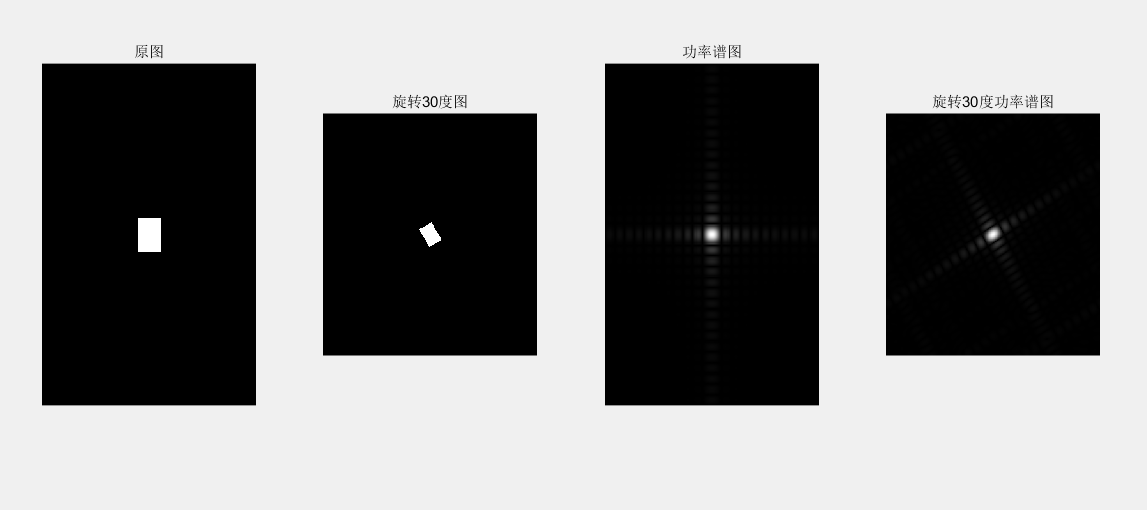## （2）InImg=imread('d:\photo\ying.jpg');subplot(2, 2, 1); imshow(InImg); title('原彩色图');I=rgb2gray(InImg);J1=imnoise(I, 'salt & pepper',0.01);subplot(2, 2, 2); imshow(J1); title('椒盐噪声污染图');[f1, f2]=freqspace([199 200],'meshgrid');        %产生等间隔采样的频率响应R=sqrt(f1.^2+ f2.^2);                   %计算功率谱Hd=ones([199 200]);Hd(R>0.5)=0;                                   %产生理想的带通滤波器，频率带宽[0.1 0.5]win= fspecial('gaussian',[199 200],2); %采用高斯函数生成同样大小的窗函数win= win./max(win(:));                       %窗口函数归一化H=fwind2(Hd,win);                           %利用窗口函数产生实际的带通滤波器 subplot(2, 2, 3); freqz2(H);ff= imfilter (J1,H);subplot(2, 2, 4); imshow(ff);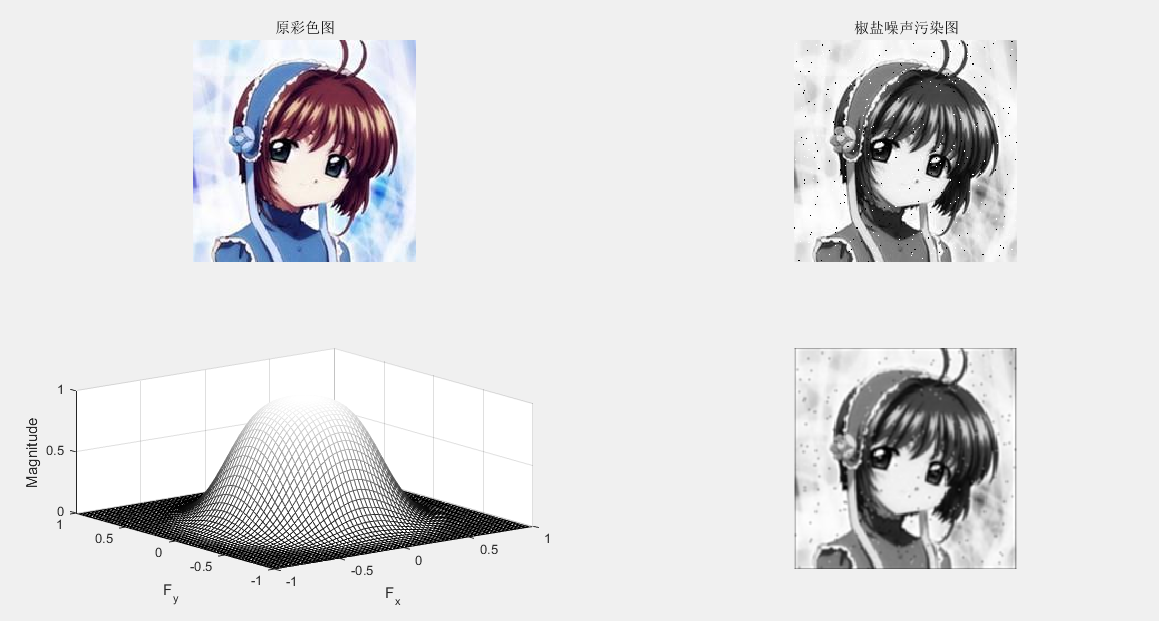## 3、图像复原

J = WIENER2(I,[M N]): 对灰度图像实施维纳自适应滤波 ，[M N]像素领域大小
C=conv2(A,B) : 计算矩阵 A B 的卷积

InImg=imread('d:\photo\yng.jpg');
I=rgb2gray(InImg);
J1=imnoise(I, 'gaussian');
B1=[0 0.2 0;0.2 0.2 0.2; 0 0.2 0];   %平滑滤波器
H = fspecial('average',[5 5]);
Blurred1 = imfilter (J1,H);
H = fspecial('gaussian',[5 5]);
Blurred2 = imfilter (J1,H);
K = wiener2(J1,[5 5]);
subplot(1, 5, 1); imshow(J1) ; %显示噪声图像
title('高斯噪声图像');
subplot(1, 5, 2); imshow(Blurred1) ; %显示均值滤波图像
title('均值滤波图像');
subplot(1, 5, 3); imshow(Blurred2) ; % 显示高斯滤波图像
title('高斯滤波图像');
subplot(1, 5, 4); imshow(K);% 显示维纳滤波器滤波图像
title('维纳滤波器滤波图像');
C1=conv2(J1,B1, 'same'); %对加噪后的图像平滑滤波
C1=mat2gray(C1); %将矩阵转换为灰度图像
subplot(1, 5, 5); imshow(C1);% 显示平滑图像
title('平滑图像');4、图像压缩

clear
close all;  %定义HufData/Len为全局变量的结构体
global HufData;
global Len;
disp('计算机正在准备输出霍夫曼编码结果,请耐心等待……'); %原始码字的灰度
a=imread('d:/photo/hua.jpg');
a=rgb2gray(a);
figure;
subplot(1,2,1); imshow(a);
%取消坐标轴和边框
axis off
box off
title('Tokyo Towers','fontsize',13);
subplot(1,2,2);
axis off
box off
imhist(a);
title('图像灰度直方图','fontsize',13);%图像的灰度统计
GrayStatistics=imhist(a);
GrayStatistics=GrayStatistics';
GrayRatioo=GrayStatistics/sum(GrayStatistics);
GrayRatioNO=find(GrayRatioo~=0);
GrayRatioyes=find(GrayRatioo==0);
Len=length(GrayRatioNO);  %初始化灰度集，防止系统随即赋予其垃圾值
GrayRatio=ones(1,Len);
for i=1:Len
GrayRatio(i)=GrayRatioo(i);
end
GrayRatio=abs(sort(-GrayRatio));
for i=1:Len
HufData(i).value=GrayRatio(i); %将图像灰度概率赋予结构体
end  % 霍夫曼编码/霍夫曼编码

HuffmanCode(Len); %输出码字
lavg1=0;
for i=1:Len
tmpData=HufData(i).code
str='';
for j=1:length(tmpData) ;
str=strcat(str,num2str(tmpData(j)));
disp(strcat('a','= ',str));
end
len1=length(tmpData) ;
lavg1=lavg1+GrayRatio(1,i)*len1;

end
ratio_delet=8/lavg1;
str='';
str=strcat(str,num2str(ratio_delet));

disp(strcat('压缩率','= ',str))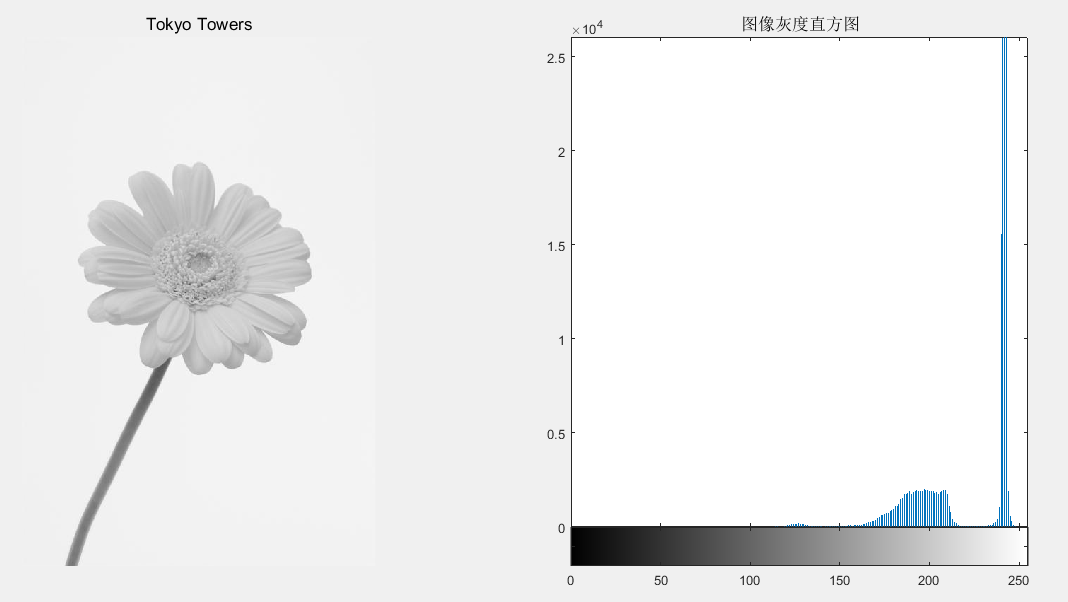## 5、图像分割（边缘提取）

### 1）图像几何纠正、边缘检测

B=imresize(A,m,type): 图像大小调整

B=imrotate(A,angle,method): 图像旋转调整

InImg=imread('d:\photo\tail.bmp');
I=rgb2gray(InImg);
B1=imresize(I,1.5, 'nearest');
B2=imresize(I,1.5, 'bilinear');
C1= imrotate (I,45, 'nearest');
C2= imrotate (I,45, 'bilinear');
subplot(231);imshow(I) ;%显示原始图像于
title('原始图像');
subplot(232); imshow(B1) ;%显示调整图像(最近邻插值)
title('最近邻插值图像');
subplot(233); imshow(B2) ;% 显示调整图像(双线性插值)
title('双线性插值图像');
subplot(234); imshow(C1);% 显示旋转图像(最近邻插值)
title('旋转最近邻插值图像');
subplot(235); imshow(C2);% 显示旋转图像(双线性插值)
title('旋转双线性插值');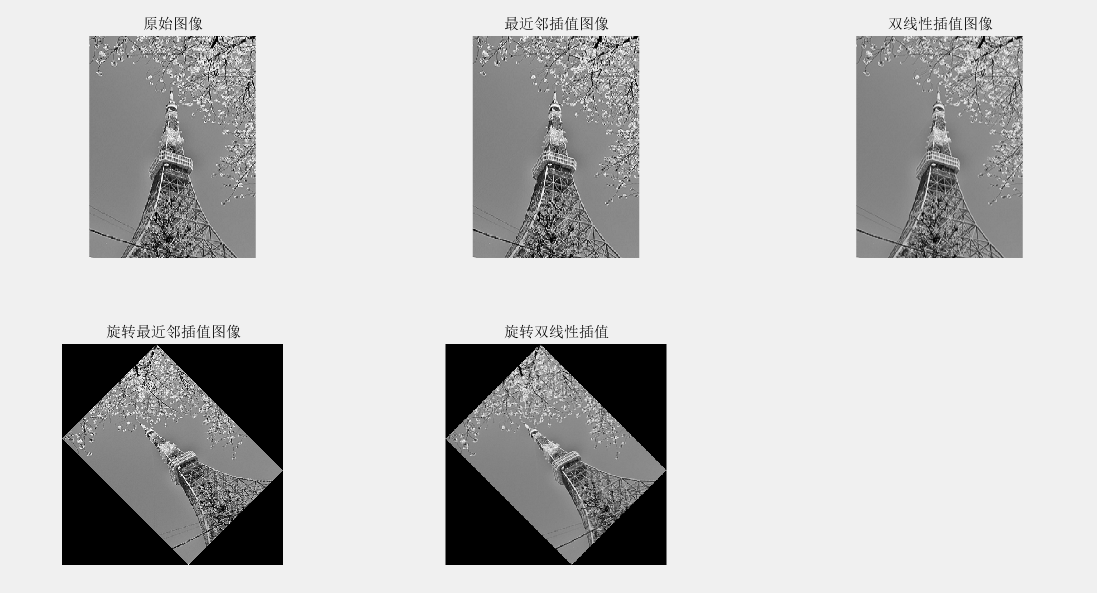2）边缘提取

BW=edge(I,method,thresh): 检测灰度图像的边缘

InImg=imread('d:\photo\ying.jpg');
I=rgb2gray(InImg);
BW1= edge (I, 'sobel',0.1);%sobel边缘检测器
BW2= edge (I, 'prewitt',0.1);%prewitt边缘检测器
BW3= edge (I, 'roberts',0.1);%roberts边缘检测器
BW4= edge (I, 'log',0.01,2.0);%log边缘检测器
subplot(221); imshow(BW1) ;% 显示边缘图像
title('sobel边缘图像');
subplot(222); imshow(BW2) ;%显示边缘图像
title('prewitt边缘图像');
subplot(223); imshow(BW3) ;% 显示边缘图像
title('roberts边缘图像');
subplot(224); imshow(BW4) ;% 显示边缘图像
title('log边缘图像');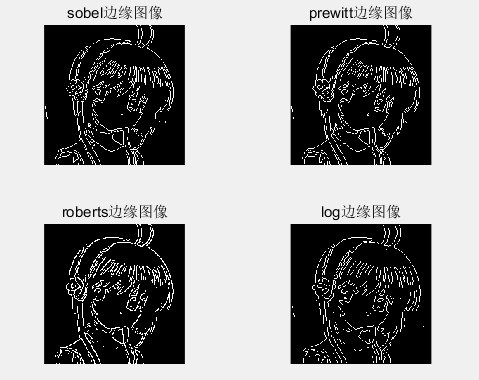## 边缘提取05-12
02-22
06-181万+
12-272万+
08-229519
12-256432
05-072130
04-10
07-091万+
©️2020 CSDN 皮肤主题: 大白 设计师:CSDN官方博客